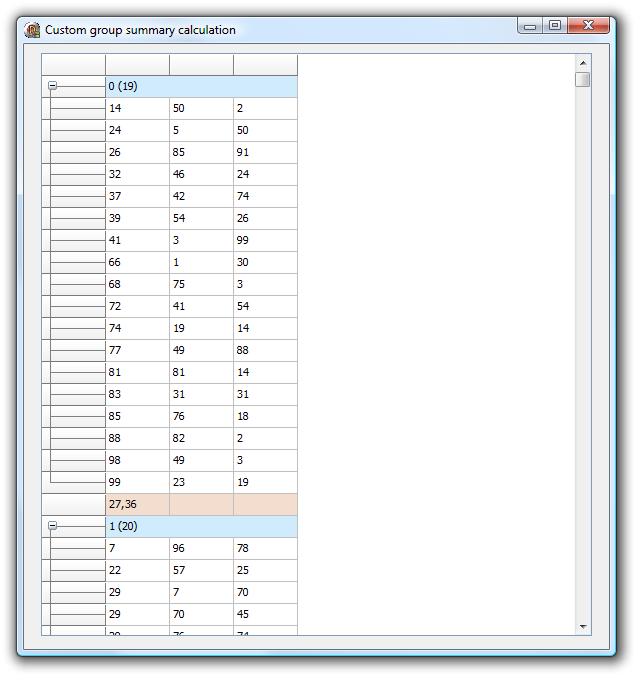Example 70 : Custom group calculationIf there is a need for a special group calculation that is not available in the standard group calculation functions, the method grid.GroupCustomCalc can be used. For each group in the grid, this will trigger the event grid.OnGroupCalc(Sender: TObject; ACol, FromRow, ToRow: Integer; var Res: Double);

The meaning of the parameters is:

ACol : column to perform calculation for FromRow: first row in the group ToRow: last row in the group Res: variable parameter to use to set the result

In this sample, the grid is initialized with random number, is grouped on column 1 and for the first column in the grouped grid the standard deviation is calculated:

The code to achieve this is here:

ToRow: Integer; var Res: Double);
var
i: integer;
d, m, sd: double;
begin

// calculate mean
m := 0;
for i := FromRow to ToRow do
begin
end;

m := m / (ToRow - FromRow + 1);

sd := 0;
for i := FromRow to ToRow do
begin
sd := sd + sqr(advstringgrid1.Floats[ACol,i] - m);
end;

sd := sd / (ToRow - FromRow);
Res := sqrt(sd);
end;

procedure
TForm1.FormCreate(Sender: TObject);
var
i: integer;
begin